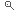PORTALE DELLA DIDATTICACERCA KEYWORD

#### Kinetic models of multi-agent systems

Reference persons ANDREA TOSIN

Research Groups Metodi e modelli matematici per sistemi complessi

Thesis type MASTER THESIS, MODELING, RESEARCH THESIS, THEORETICAL

Description *** NOTICE *** Those interested in this proposal of MSc thesis should contact me by email. Please, do not send requests from Portale della Didattica.

In this project, we are interested in the modelling of multi-agent systems by means of Boltzmann-type kinetic equations. Kinetic models consist in integro-differential equations describing the time evolution of the statistical distribution of the microscopic states of the agents. A plethora of different applications can be studied in this setting, among which we mention for instance:
- vehicular traffic, where the agents are the vehicles with microscopic state given by their position and speed along a road (or a road network);
- pedestrian traffic, where the agents are the pedestrians with microscopic state given by their position and velocity normally in a two-dimensional domain;
- opinion dynamics, where the agents are individuals with microscopic state given by their opinion about a certain issue;
- wealth redistribution dynamics, where the agents are economical traders with microscopic state given by their wealth;
- transmission of infectious diseases, where the agents are individuals with microscopic state given by the viral load they carry.

Kinetic equations allow one to model the interactions among the agents responsible for the variation in time of the microscopic state of the latter. From this basis, they make it possible to describe the aggregate evolution of the macroscopic characteristics of the system and, in particular, to study the so-called emerging trends, namely the collective manifestations arising spontaneously on a large scale from the individual interactions. The models that we propose are inspired by the Boltzmann equation, where the collisions among gas molecules are described by an integral collision operator. The study of the mathematical properties of this operator is at the basis of the identification of the asymptotic statistical distributions, which characterise the previously recalled emerging trends.

Apart from the specific application, the project encompasses three main methodological aspects: modelling, analysis and numerical simulation, which can be more or less developed according to the student's taste.

Required skills - Elementi di equazioni differenziali alle derivate ordinarie e parziali;
- Equazioni della fisica matematica;
- Elementi di teoria della probabilità;
- Modelli di trasporto e teorie cinetiche;
- Analisi numerica ed elementi di programmazione

Notes References:
- L. Pareschi, G. Toscani. Interacting Multiagent Systems: Kinetic equations and Monte Carlo methods. Oxford University Press, 2013.

Deadline 01/12/2021      PROPONI LA TUA CANDIDATURA